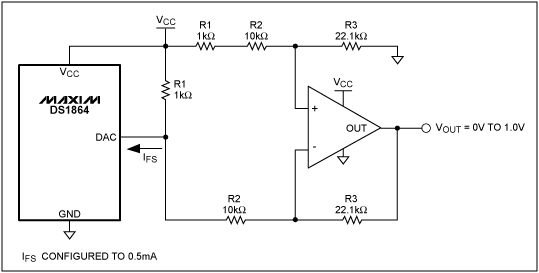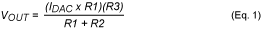# How to Use the DS1864's Current DACs as Voltage DACs

### Abstract

This article describes how to convert the current DACs in the DS1864 SFP laser controller/monitor to a voltage output by using standard components.

### The Current DACs

The DS1864 contains two 8-bit current-sink DACs with 1.5mA or 0.5mA selectable full-scale range. The DAC outputs must be at a voltage level between 0.7V and the DS1864's VCC.

### Current-to-Voltage Conversion

An operational amplifier in differential amplifier configuration is used to convert the 0.5mA full-scale current-mode output to 1V full-scale voltage-mode output (Figure 1).### Compact Solution

Using a chip-scale packaged op amp and 0201 resistors will provide the most compact solution. The MAX4233 is a two-channel op amp available in 1.5mm x 2mm 10-bump UCSP™.

### Output Voltage Calculation

The output voltage is calculated in Equation 1.Using the values shown in the Figure 1 drawing, the maximum output voltage is 1.005V when the DAC current is 0.5mA. To minimize offset and gain errors, 1% tolerance resistors should be used. Further, R1 should be selected so that the voltage at the DAC pin never falls below 0.7V.